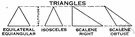equilateral triangle

Also found in: Thesaurus, Encyclopedia, Wikipedia.
Related to equilateral triangle: isosceles triangle, scalene triangle
ThesaurusAntonymsRelated WordsSynonymsLegend:
 Noun 1equilateral triangle - a three-sided regular polygon  equiangular triangleregular polygon - a polygon with all sides and all angles equaltriangle, trigon, trilateral - a three-sided polygondelta - an object shaped like an equilateral triangle
References in classic literature ?
Take for example an equilateral Triangle -- who represents with us a Tradesman of the respectable class.
In the first course, there was a shoulder of mutton cut into an equilateral triangle, a piece of beef into a rhomboides, and a pudding into a cycloid.
It happened that the Lancashire Queen, the shore at Turner's Shipyard, and the Solano Wharf were the corners of a big equilateral triangle.
If the courts is to be chalked out in white, I can draw a line so straight that you could hardly keep yourself from erecting an equilateral triangle on it.
And a few degrees to the west, 5 and 6 Lyncis form a line pointing to a nearly perfect equilateral triangle bounded by 2 Lyncis and 37 and 40 Camelopardalis.
Contract awarded for 60xxn23-supply of retro reflective road warning boards with 2mm alluminium for manned level crossing gates of size 900mm equilateral triangle with 600 x 200 x 3.
It can be constructed by first drawing an equilateral triangle and then adding more equilateral triangles at the centre of each side.
Table 1 Sample Polygon Observation Table Polygon Odd or even # Degree of of vertices rotational symmetry Equilateral triangle Odd 3 Square Even 4 Regular pentagon Odd 5 Regular hexagon Even 6 Regular septagon Odd 7 Regular octagon Even 8 Polygon Degrees per Sets of turn parallel sides Equilateral triangle 120 0 Square 90 2 Regular pentagon 72 0 Regular hexagon 60 3 Regular septagon [approximately equal to] 51.
The pyramid takes the shape of an equilateral triangle when a population is increasing.
The equilateral triangle, frequently used in Islamic patterns for its tessellating shape, is a symbol in Christian tradition of the Trinity, with three sides and three equal angles.
Then, ask students to draw an equilateral triangle on a large sheet of paper.
The standard-size products are offered in five different sizes ranging from a small 3,6m equilateral triangle up to a 5m x 5m square.

Site: Follow: Share:
Open / Close